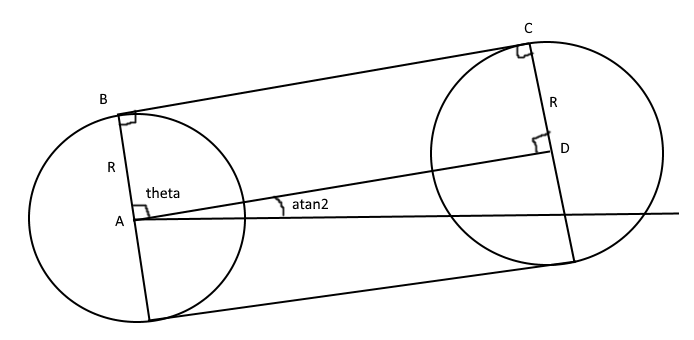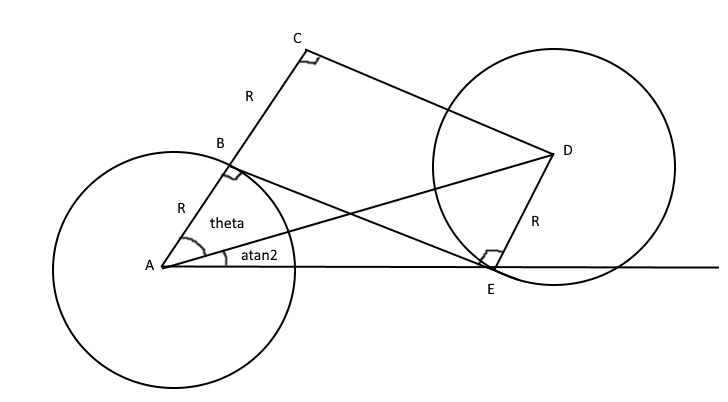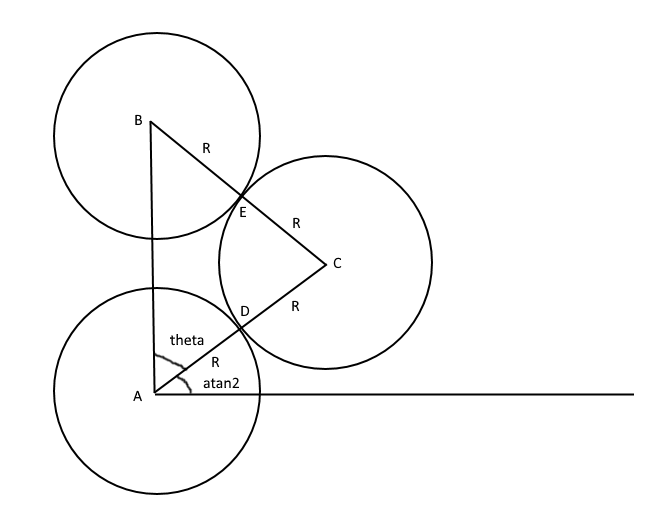# How to make Dubins Paths in Unity with C# code

## 2. Basic Dubins Paths

This will be a super short introduction to Dubins paths. Other people have written longer descriptions of Dubins paths, so if you want to read more you should maybe read this: A Comprehensive, Step-by-Step Tutorial to Computing Dubins Paths.

So how do we calculate Dubins paths? Let's repeat the definition from Wikipedia:

In geometry, the term Dubins path typically refers to the shortest curve that connects two points in the two-dimensional Euclidean plane (i.e. x-y plane) with a constraint on the curvature of the path and with prescribed initial and terminal tangents to the path, and an assumption that the vehicle traveling the path can only travel forward. If the vehicle can also travel in reverse, then the path follows the Reeds-Shepp curve.

So the car can only drive forward with constant speed. According to the inventor of Dubins paths, Lester Dubins, there are a maximum of 6 paths you need to calculate to find the shortest distance from A to B if you are a car driving forward with constant speed. Depending on the position of the car and the target we want to reach, there may be fewer than 6 paths.

The 6 paths are described by the following path segments: drive Right, drive Left, and drive Straight. So we end up with the following combinations: RSR, LSL, RSL, LSR, LRL, RLR.

To be able to construct these 6 paths we first have to add 4 circles: 1 circle 90 degrees to the right of the car, 1 circle 90 degrees to the left of the car, 1 circle 90 degrees to the right of the target car, and 1 circle 90 degrees to the left of the target car. It will look like this:Now we need to connect these circles with so-called tangent lines. A tangent line to a circle is perpendicular to the center of the circle. There are 2 different groups of tangent lines: Outer tangent and Inner tangent. The difference is that the inner tangent lines cross the center line that connects the center points of the circles.Now we can group the 6 paths into 3 different groups, and each group needs a different calculation. RSR and LSL will always use an outer tangent line, RSL and LSR will always use an inner tangent line, and LRL and RLR will not have a tangent line at all, but 2 tangent coordinates that connects with a third circle.

One good thing that will make things less complicated is that each path will always have the same tangent line. For example, RSR will always use the upper outer tangent line, no matter the orientation of the car and the target:So let's learn how to calculate the tangent lines and coordinates.

### Outer tangent lines

Calculating the outer tangent lines are easy if the circles have the same radius. This is what we have:What we have is the center coordinates of the both circles, and we want to figure out coordinate B and C. We begin with B. The angle theta is always 90 degrees if the circles have the same radius. But if the circles are not in line (are not at the same "height") we also have to calculate the angle atan2 to compensate for the rotation.

When we have the angle theta (and modified it with atan2), we can calculate the first tangent coordinate B like this: Bx = Ax + R * cos theta and By = Ay + R * sin theta.

To get the other tangent point C, we can use the fact that the center line between the circles have the same direction and length as the tangent line. This vector is D-A, and then we just add this vector to the coordinate B to get the second tangent point C.

To get the bottom tangent line, we just repeat the above calculations, but we add pi to the angle theta. The reason is that the bottom tangent is on the opposite side of the upper tangent line, and pi radians is the same as 180 degrees. So now we can find the tangent coordinates belonging to RSR (top tangent line) and LSL (bottom tangent line)!

### Inner tangent lines

Alright, 2 paths down, 4 to go. Now we will figure out how to calculate the tangent lines belonging to LSR and RSL, which are the inner tangents. This is what we have:We are interested in finding the coordinates B and E. We have the coordinates A and D (center coordinates of each circle) as well as the circle radius R.

With the help of the center coordinates of each circle we can calculate the distance between the circles, which we call D. If we know the distance between A and D and the radius of the circle, we can calculate theta with cosine: theta = acos(2R / D). And as when we calculate the outer tangents, we have to compensate for the fact that the circles don't have the same position by calculating the angle atan2.

Now (as with the outer tangent lines) we can calculate the first tangent point B. But the difference now is that we can't calculate the direction of the tangent by just using the vector between the center of the circles. So to get the direction of the tangent we also have to calculate coordinate C by using the same math as when we calculated B, but instead of R we use 2R.

The line CD and the tangent line BE have the same direction, and since we now know both C and D we can calculate the direction as we did with the outer tangent line. Then we just add the direction to B to get the final tangent coordinate E.

Now we know the tangent line RSL (from the top of the right start circle to the bottom of the left goal circle) and LSR (bottom-to-top tangent).

### LRL and RLR tangent coordinates

The last path curves are a little special, because now we have to add a third circle with the same size as the other two circles. It looks like this:As before we have the center position of each circle: A and B. We also have the circle radius R. We are interested in the coordinates D and E.

As you might think, we first have to calculate the angle theta. To get theta we have to use the law of cosines. And if D is the distance between the center position of the circles (not to be confused with tangent coordinate D), the law of cosines becomes: cos theta = (D^2 + (4R)^2 - (4R)^2) / (2 * D * 2* R) = D / 4R. And as before we have to compensate for the fact that the circles don't have the same position by calculating angle atan2.

With theta we can get the center coordinate of the third circle in the same way as we before calculated the first tangent position of the inner and outer tangent: Cx = Ax + 2R * cos theta and Cy = Ay + 2R * sin theta.

Now E is on the same line as BC and D is one the same line as AC. So we can calculate the direction between BC and AC and normalize it. To get tangent coordinates we just multiply the direction with the radius R and add it to the center coordinate C of the third circle.

And that's it! The boring math part is over. Well, you'll have to learn on your own how to calculate arc lengths and how to iterate over the path with a numerical method, but that's easy. Now we will create something looking like this in the next part (the white line is the shortest of the Dubins paths):